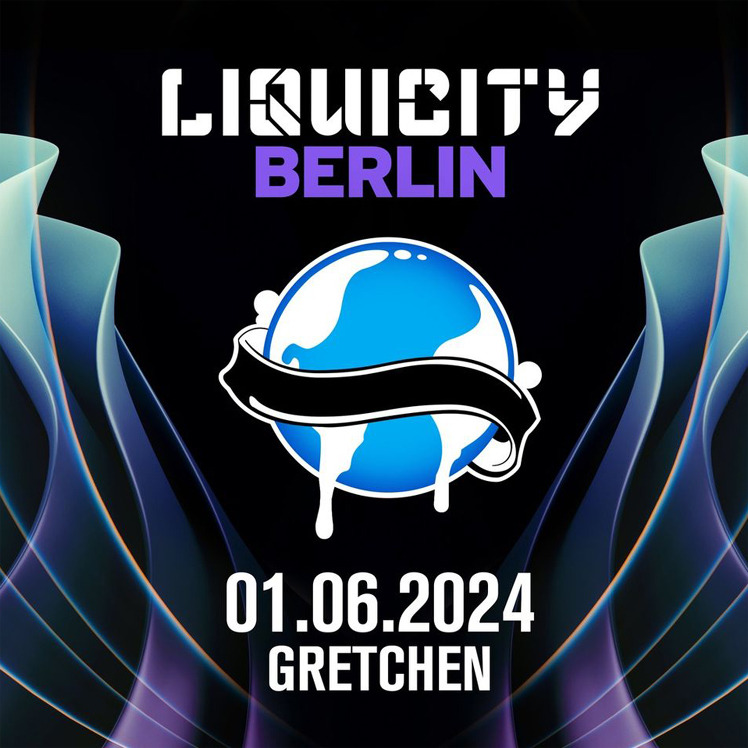### BERLIN:FM

< BACKDi. 28.11. Mi. 29.11. Do. 30.11. Fr. 01.12. Fr. 01.12. Sa. 02.12. Mo. 04.12. Di. 05.12. Do. 07.12. Fr. 08.12. Fr. 08.12. So. 10.12. Di. 12.12. Di. 12.12. Mi. 13.12. Do. 14.12. Fr. 15.12. Sa. 16.12. So. 17.12. Mo. 18.12. Di. 19.12. Sa. 30.12. So. 31.12. Fr. 05.01. Mi. 10.01. Sa. 20.01. Fr. 26.01. Fr. 26.01. Di. 30.01. Mi. 31.01. Fr. 02.02. Sa. 03.02. Mi. 07.02. Sa. 10.02. Sa. 10.02. So. 11.02. Mo. 12.02. Fr. 16.02. So. 25.02. Mo. 26.02. Di. 27.02. Mi. 28.02. Fr. 01.03. Sa. 02.03. Mo. 04.03. Di. 05.03. Sa. 09.03. So. 10.03. Di. 12.03. Mi. 13.03. Do. 14.03. So. 17.03. Mo. 18.03. Mi. 20.03. Mi. 20.03. Do. 21.03. Sa. 23.03. Fr. 05.04. Sa. 06.04. Mo. 08.04. Di. 09.04. Mi. 10.04. Sa. 13.04. Di. 16.04. Fr. 19.04. Di. 23.04. Mi. 24.04. Do. 25.04. So. 28.04. Fr. 03.05. Sa. 04.05. Mi. 29.05. Sa. 01.06.
Drum'n'Bass Sa. 01.06.2024
Doors: 23.00

## Liquicity presents: LIQUICITY BERLIN

Liquicity is going to touch down in Berlin for the very first time in history!
It might still seem far away, with the date of this debut being 1st of June 2024, but it just did not feel right to keep this from you any longer   Berlin will be the 3rd German city Liquicity is going to conquer and, if the events in its predecessors Heidelberg & Cologne are any indication, this one will be just as epic
Now it’s hard to say this as you’re probably already preparing to buy tickets, but they are not available just yet! However, to make sure you are the very first to snatch one of these limited tickets when they do, drop your email address at https://liquicity.com/berlin-signup & get notified

Text & Artwork: @ Liquicity18 - 24 € Online Ticket